# Trigonometry Formula, Tricks And Questions

## Trigonometry Formulas, Tricks & Questions

Trigonometry is an important part of the Quantitative Aptitude section that gives a tough time to students with its complex formulas and questions. Trigonometry is a major branch of mathematics that deals with the angles and lengths of a right-angled triangle. The application of trigonometry is done in astronomical studies and other science fields. There are a total of 6 trigonometric functions namely Sin, Cos, Tan, Sec, Cosec, and Cot. There are trigonometric ratios that help to derive the current length and angle. All the Trigonometry formulas, tricks and questions in trigonometry revolve around these 6 functions. Aspirants can check out the details of Trigonometry including the formulas, tricks and questions.

Questions from the Trigonometry topic are asked in various competitive examinations such as SSC, Railway etc. In this post, we are providing you with the Trigonometry notes useful for the examinations. It will help you memorize basic formulas of Trigonometry.

### Important Trigonometric Ratio Identities

In order to get hold of the basic concepts of trigonometry, you must learn all the important trigonometric ratio and their identities.

Trigonometric Ratios

To study different trigonometric ratio functions,  we will use a right-angled triangle. Suppose ABC is a right-angled triangle with angle A = 90°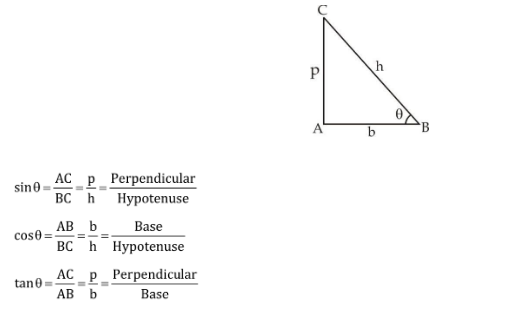Relations between Trigonometric Ratios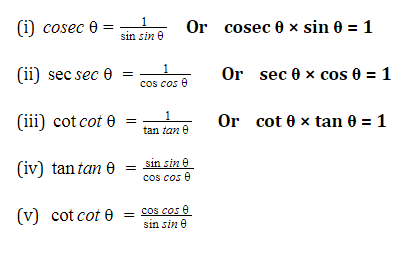Value of Trigonometric Ratios in Different Quadrants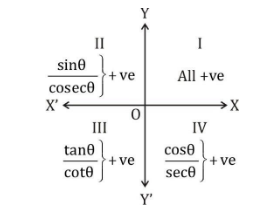Different Values of Specific Angle of Trigonometric Ratio

You must learn the following table to solve the question based on the trigonometrical ratio of angle 0°, 30°, 45°, 60°

###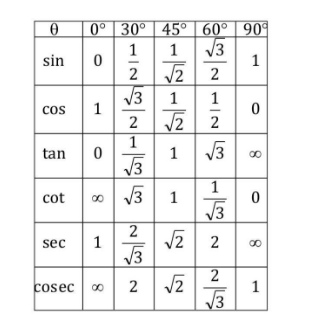Trigonometry Formula: Relation Between Square Of Different Types Of Trigonometric ratios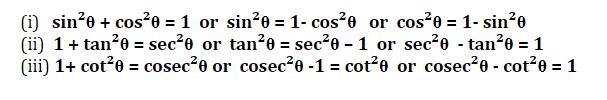Important Concept to Solve a Specific Type of Question

If A + B = 90°

Results that are true always :

(i)  sin A. sec B = 1 or  sin A = cos B

(ii) cos A. cosec B = 1 or  sec A = cosec B

(iii) tan A. tan B = 1  or tan A = cot B

(iv) cot A. cot B = 1

(v)  sin²A + sin² B = 1

(vi) cos² A + cos² B = 1

Important Trigonometry Formula for Sum and Difference Of Two Angles

(1) sin (A+B) =sinA. cosB + cosA sinB

(2) sin(A – B) =sinA. cosB – cosA sinB

(3) cos(A+B) =cosA. cosB – sinA sinB

(4) cos(A-B) = cosA. cosB+sinA sinB

(5) 2 sinA.cosB = sin(A+B)+sin (A-B)

(6) 2 cosA. sinB = sin(A+B)-sin (A-B)

(7) 2 sinA. sinB = cos(A-B)-cos(A+B)

(8) 2 cosA.cosB = cos(A+B)+cos(A-B)

(9) sin²A-sin²B = sin(A+B). sin(A-B)

(10) cos²A-cos²B = cos(A+B).cos (A-B)

Trigonometry Formulas For Tangent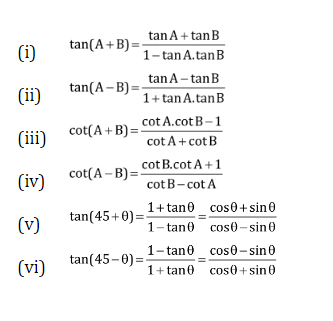### Important Results for Trigonometry

• If A + B + C = 180°

Then, tan A + tan B + tan C = tan A. tan B. tan C

• If A + B + C = 90°

Then,  cot A + cot B + cot C = cot A cot B cot C

• If (a) sin θ + cosec θ = 2

Then,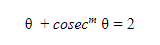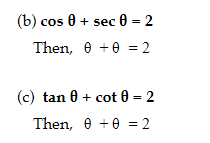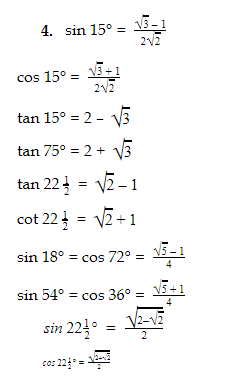### Trigonometry: Maximum & Minimum Value

Maximum & Minimum Value

Minimum                           Maximum

• sin θ, cos θ [odd power]             –1                                         +1
• sin θ, cos θ [even power]             0                                         +1
• tan θ, cot θ [odd power]             –∞                                       +∞
• tan θ, cot θ [even power]             0                                        +∞
• sec θ, cosec θ [odd power]         –∞                                       +∞
• sec θ, cosec θ [even power]        +1                                        +∞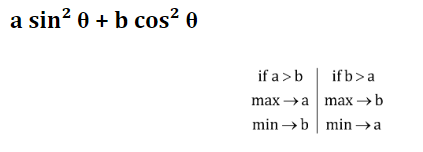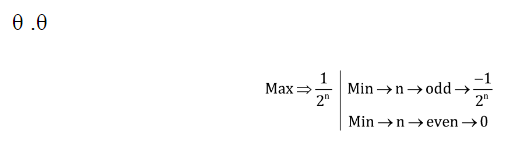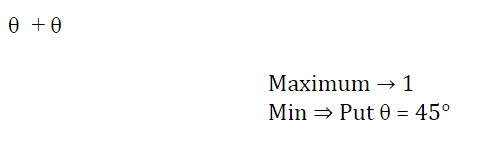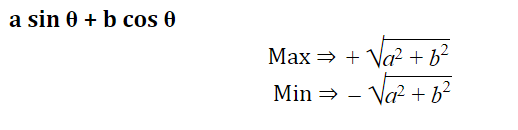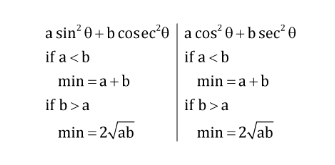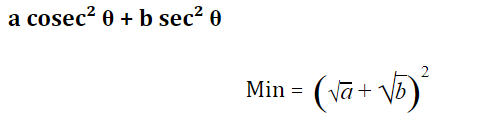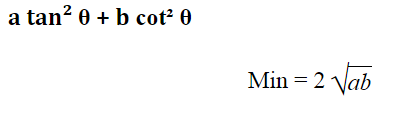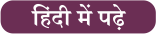For today, buy the SSC Maha Pack for just Rs. 99/- using Adda247 App Only